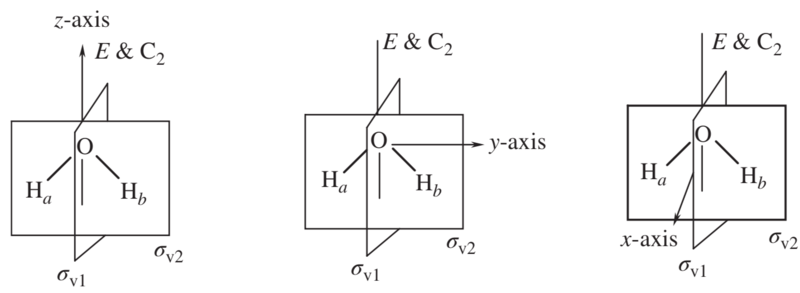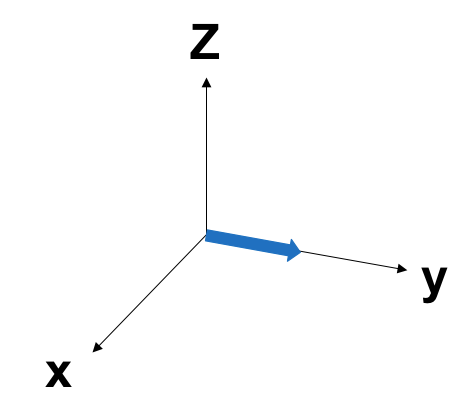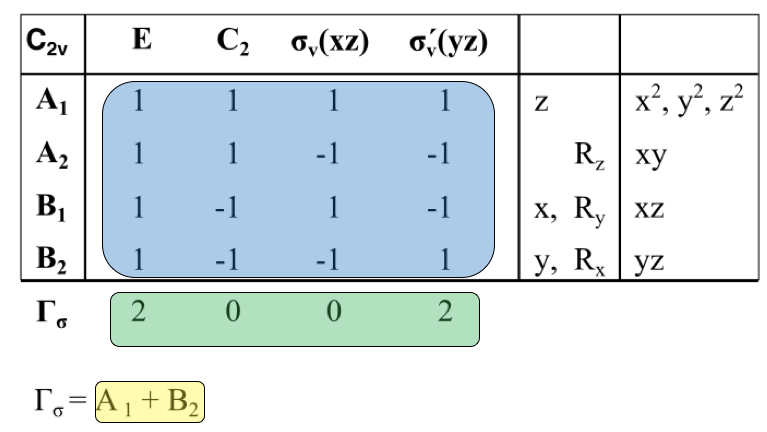# 5.03: Representations

$$\newcommand{\vecs}{\overset { \rightharpoonup} {\mathbf{#1}} }$$ $$\newcommand{\vecd}{\overset{-\!-\!\rightharpoonup}{\vphantom{a}\smash {#1}}}$$$$\newcommand{\id}{\mathrm{id}}$$ $$\newcommand{\Span}{\mathrm{span}}$$ $$\newcommand{\kernel}{\mathrm{null}\,}$$ $$\newcommand{\range}{\mathrm{range}\,}$$ $$\newcommand{\RealPart}{\mathrm{Re}}$$ $$\newcommand{\ImaginaryPart}{\mathrm{Im}}$$ $$\newcommand{\Argument}{\mathrm{Arg}}$$ $$\newcommand{\norm}{\| #1 \|}$$ $$\newcommand{\inner}{\langle #1, #2 \rangle}$$ $$\newcommand{\Span}{\mathrm{span}}$$ $$\newcommand{\id}{\mathrm{id}}$$ $$\newcommand{\Span}{\mathrm{span}}$$ $$\newcommand{\kernel}{\mathrm{null}\,}$$ $$\newcommand{\range}{\mathrm{range}\,}$$ $$\newcommand{\RealPart}{\mathrm{Re}}$$ $$\newcommand{\ImaginaryPart}{\mathrm{Im}}$$ $$\newcommand{\Argument}{\mathrm{Arg}}$$ $$\newcommand{\norm}{\| #1 \|}$$ $$\newcommand{\inner}{\langle #1, #2 \rangle}$$ $$\newcommand{\Span}{\mathrm{span}}$$$$\newcommand{\AA}{\unicode[.8,0]{x212B}}$$

## Representation

A representation is a set of matrices, each of which corresponds to a symmetry operation and combine in the same way that the symmetry operators in the group combine.1 Symmetry operators can be presented in matrices, this allows us to understand the relationship between symmetry operators through calculation from matrices. In order to understand representations, knowing matrix notions for symmetry operations is essential.

Here is some examples of symmetry operations in matrix form:

$\text { a point in space }=\left[\begin{array}{l}{x} \\{y} \\{z}\end{array}\right]\nonumber$

$E=\left[\begin{array}{lll} {1} & {0} & {0} \\ {0} & {1} & {0} \\ {0} & {0} & {1} \end{array}\right]\nonumber$

$i=\left[\begin{array}{ccc} {-1} & {0} & {0} \\ {0} & {-1} & {0} \\ {0} & {0} & {-1} \end{array}\right]\nonumber$

$\sigma_{x y}=\left[\begin{array}{lll} {1} & {0} & {0} \\ {0} & {1} & {0} \\ {0} & {0} & {1} \end{array}\right]\nonumber$

$\mathrm{C}_{\mathrm{n}}=\left[\begin{array}{ccc} {\cos \theta} & {-\sin \theta} & {0} \\ {\sin \theta} & {\cos \theta} & {0} \\ {0} & {0} & {1} \end{array}\right] \text { (setting zas the principal axis) }\nonumber$

$S_{n}=\left[\begin{array}{ccc} {\cos \theta} & {-\sin \theta} & {0} \\ {\sin \theta} & {\cos \theta} & {0} \\ {0} & {0} & {-1} \end{array}\right] \text { (setting zas the principal axis) }\nonumber$

For Cn operation, θ depends on n with the relationship of $$\theta=\frac{360}{n}$$. If the symmetry is C2, then θ would be 180° because the molecule is rotated 180°. For C3, θ would be 120°, C4 θ would be 90°, etc.

To apply a symmetry operation on an atom of a molecule, matrices can be combined to produce another operation in the group. For a C2v symmetry compound such as water shown in figure $$\PageIndex{1}$$, the operations (E, C2, σv1, σv2) can be applied on the vector (x, y, z) to find the representation. To simplify the math, a 1x1 matrix can be done by block diagonalizing for individual vector.

For example,

E(y) =  [y] = y

C2(y) = [-1] [y] = -y

σv1(y) = σxz = [-1] [y] = -y

σv2(y) = σyz =  [y] = y

In this example, if you apply identity (E) on vector y shown in figure $$\PageIndex{2}$$, you would obtain y. If you apply C2 rotation along the principal axis on y, then you would obtain -y, etc. These obtained results show the position of the vector after the symmetry operation. The coefficient of each vector after symmetry operation can be represented as Γy in character table $$\PageIndex{1}$$. This set of matrices each of which corresponds to the character of a matrix a representation. The same symmetry operation can be applied on x and z to obtain a representation for Γx and Γz.

Table $$\PageIndex{1}$$. xyz representations in C2v.

C2v E C2 σv1 σv2
Γz    
Γy  [-1] [-1] 
Γx  [-1]  [-1]Figure $$\PageIndex{1}$$. Water molecule a) z-axis b) y-axis c) x-axis.Figure $$\PageIndex{2}$$. y vector on a cartesian coordinate.

A representation can combine in the same way that the symmetry operators in the group combine, thus, the multiplication table for the matrices that represent each symmetry operation must also multiply together in the same way that the symmetry operators themselves multiply.1 Refer to the following tables.

Multiplication tables for the C2v point group, showing how the 1 × 1 matrix representations multiply together in the same way that the symmetry operations do.

C2v E C2 σv1 σv2
E E C2 σv1 σv2
C2 C2 E σv2 σv1
σv1 σv1 σv2 E C2
σv2 σv2 σv1 C2 E

Γz    
    
    
    
    

Γy  [-1] [-1] 
  [-1] [-1] 
[-1] [-1]   [-1]
[-1] [-1]   [-1]
  [-1] [-1] 

Γx  [-1]  [-1]
  [-1]  [-1]
[-1] [-1]  [-1] 
  [-1]  [-1]
[-1] [-1]  [-1] 

## Irreducible Representation and Reducible Representations

A representation can be categorized as irreducible representation and reducible representations. A character table is given with irreducible representations, which are the blue shaded part in figure 5. There are 5 rules to irreducible representations, shown in the following.

5 Rules:

1. The sum of the squares of the dimensions of the irreducible representations of a group is equal to the order of the group.

h = Σi li2

2. The sum of the squares of the characters in any irreducible representation equals h.

h = Σi [Xi (R)2]

3. The vectors whose components are the characters of two different irreducible representations are orthogonal.

ΣR [XiR * XjR] = 0, if i =/= j

4. In a given representation (reducible or irreducible) the characters of all matrices belonging to symmetry operations in the same class are identical.

The classes correspond directly to the sets of equivalent operations. Two operations belong to the same class when one may be replaced by the other in a new coordinate system that is accessible by a symmetry operation. For example, a C7 point group would have C71, C72, C73, C74, C75, C76, C77. Since cos(θ) = cos(θ), the characters associated with these matrices are the same. In this case, C71=C76, C72 = C75, C73 = C74, and C77 = E because they belong to the same class. We can simplify the C7 point group’s symmetry into 2 C71, 2C72, 2C73, and E because there are two operations belonging to the same class.

5. The number of irreducible representations of a group is equal to the number of classes in the group.Figure $$\PageIndex{3}$$. a) Blue shaded part: irreducible representation b) Green shaded part: reducible representation c) Yellow shaded part: Reducing reducible representations into irreducible representation

The green shaded part in figure $$\PageIndex{3}$$ is a the reducible representation that is found based on number of unmoved molecule after a symmetry operation. For example, if we are looking at Γσ of C2v symmetry molecule such as water from figure $$\PageIndex{3}$$, we would focus on the number of unmoved σ bonds after the symmetry operation. In a water molecule, there are two s bonds which are the two O-H bonds. If we apply E, both of the bonds don’t move, so the reducible representation would be 2 because each unmoved σ bonds contribute to 1 reducible representation. If we apply C2 operation on it, both bonds would move, where the O-H bonds would switch places. This means that there are zero unmoved σ bonds, so the reducible representation would be zero as shown in figure $$\PageIndex{3}$$ b.

The yellow shaded part in figure $$\PageIndex{3}$$ is the reduction of reducible representations into irreducible representations. This can be done by using the formula, $$\displaystyle \mathrm{n}_{\mathrm{i}}=\frac{1}{h} \sum_{\mathrm{N}} \mathrm{X}_{\mathrm{R}} \mathrm{X}_{\mathrm{L}}$$, where ni = # of times the irreducible representation occurs in the reducible representation, N = the coefficient in front of each symmetry element symbol (shown on the top row of the character table), h = order of the group (sum of the coefficients N), XR, XI = characters of the reducible and irreducible representations.

#### Reference

1.     Pfennig, Brian (2015). Principles of Inorganic Chemistry. Hoboken, New Jersey: John Wiley & Sons, Inc.. pp. 195–202. ISBN 978-1-118-85910-0.

5.03: Representations is shared under a CC BY-SA license and was authored, remixed, and/or curated by LibreTexts.